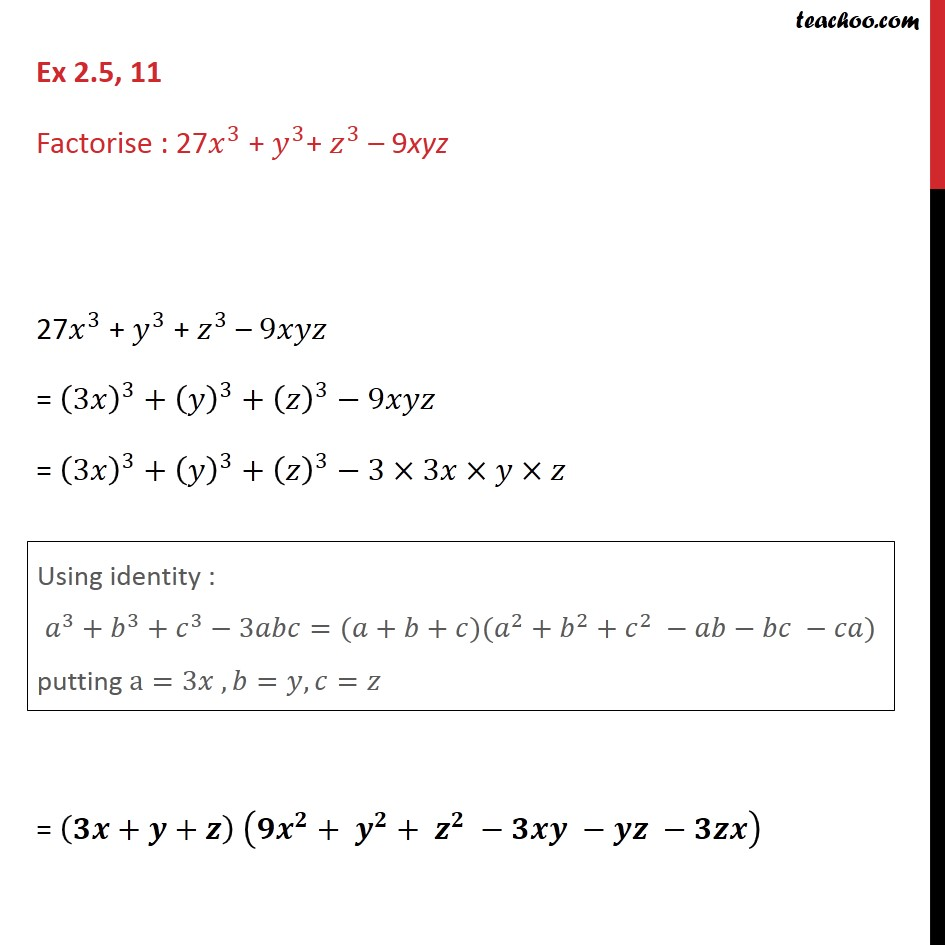Learn all Concepts of Polynomials Class 9 (with VIDEOS). Check - Polynomials Class 91. Chapter 2 Class 9 Polynomials
2. Serial order wise
3. Ex 2.5

Transcript

Ex 2.5, 11 Factorise : 27 3 + 3 + 3 9xyz 27 3 + 3 + 3 9 = 3 3 + 3 + 3 9 = 3 3 + 3 + 3 3 3 = + + + +

Ex 2.5

Chapter 2 Class 9 Polynomials
Serial order wise